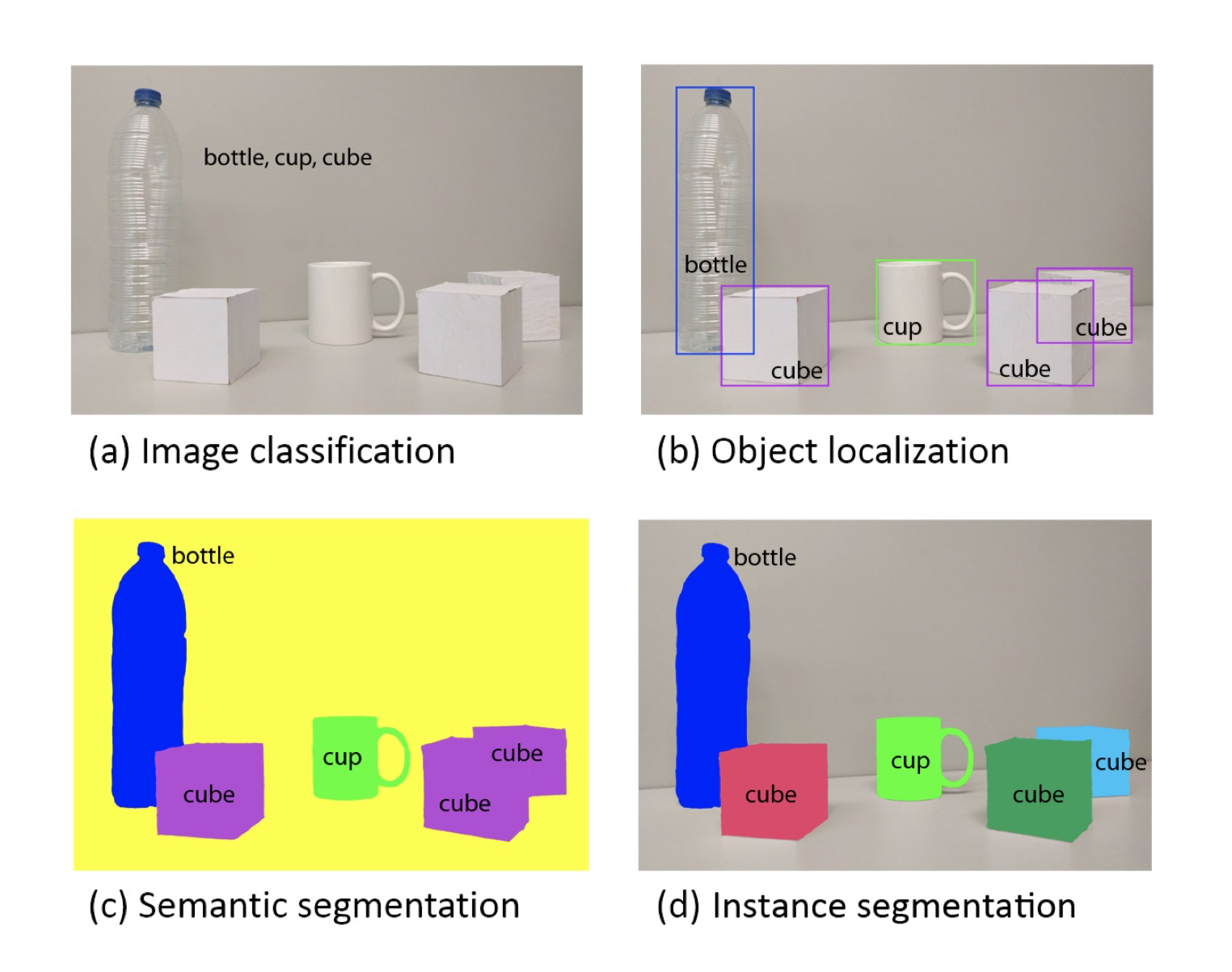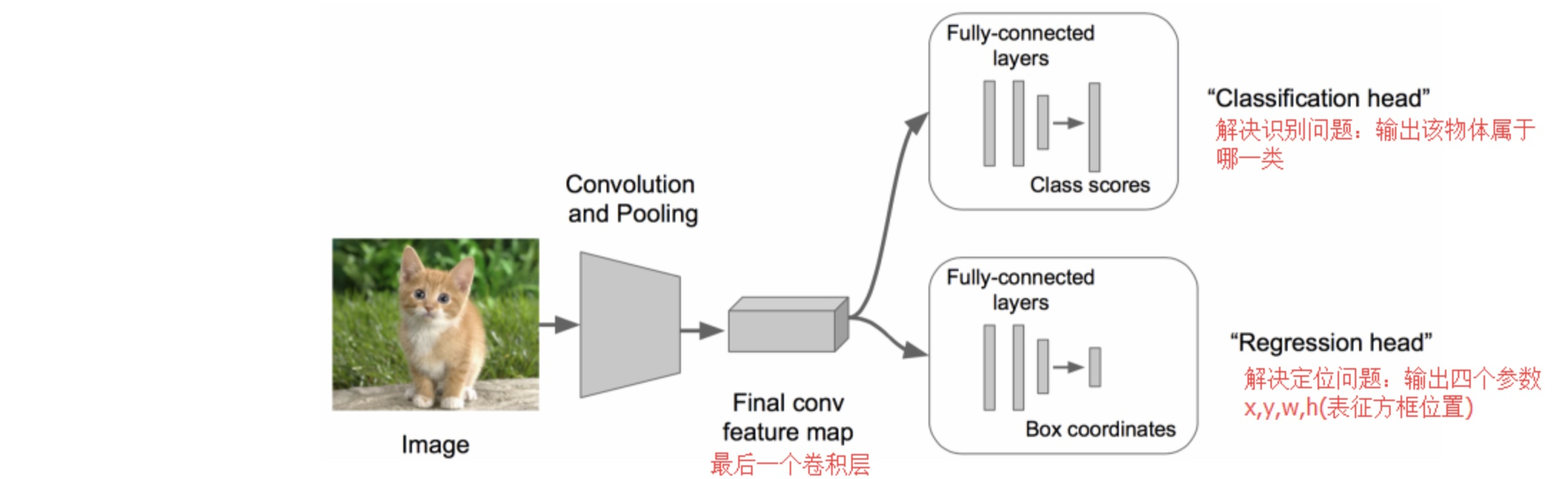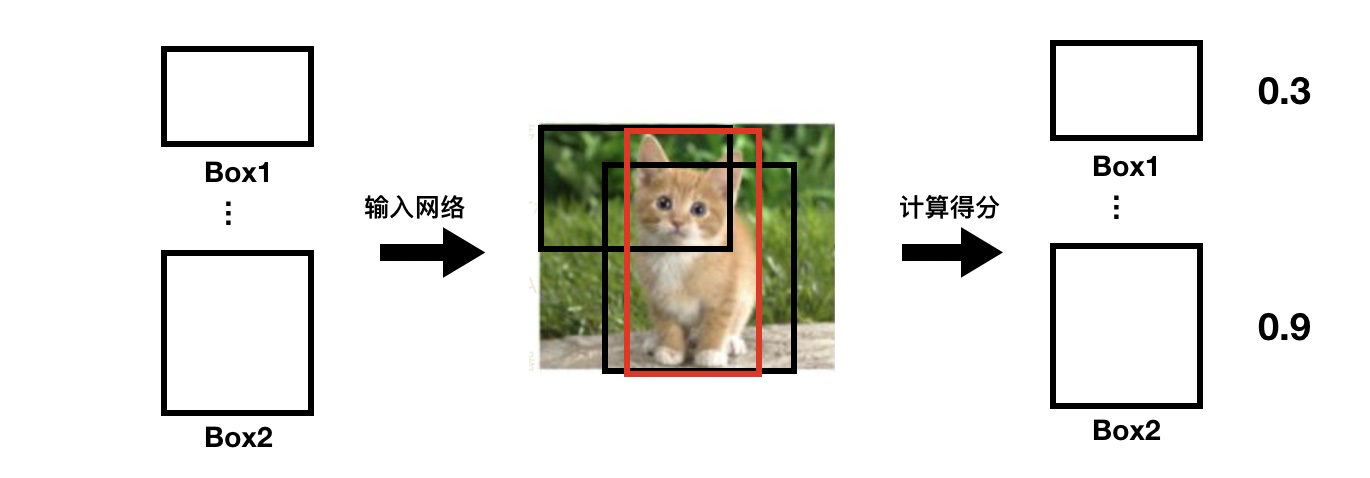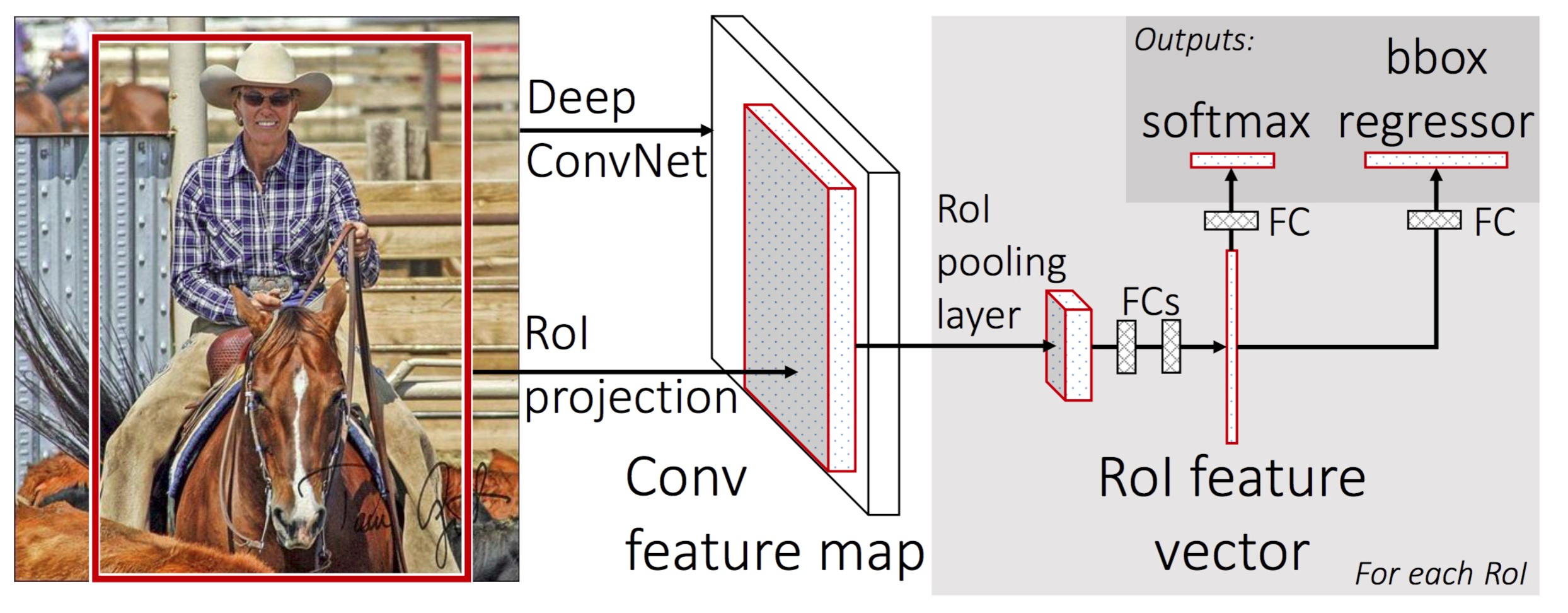http://blog.afuture.me AFuture's Blog 2020-05-04T08:28:06.462Z https://github.com/jpmonette/feed Life & Knowledge http://blog.afuture.me/images/avatar.png http://blog.afuture.me/favicon.ico All rights reserved 2020, AFuture's Blog <![CDATA[求积分]]> http://blog.afuture.me/post/WmYc9YkLS/ 2019-11-17T08:55:35.000Z Question

$calculate \int_{0}^{\frac{\pi}{2}}\frac{x\ln{\cos(x)}}{\tan x}dx$

]]>
Question

$calculate \int_{0}^{\frac{\pi}{2}}\frac{x\ln{\cos(x)}}{\tan x}dx$

\begin{aligned} I&=\int_{0}^{\frac{\pi}{2}}\frac{x\ln{(\cos x)}}{\tan x}dx=\int_{0}^{\frac{\pi}{2}}x\ln{(\cos x)}d(\ln(\sin x))\\ &=x\ln{(\cos x)}\ln(\sin x)\left|_{0}^{\pi/2}\right.-\int_{0}^{\frac{\pi}{2}}\ln{(\sin x)}d(x\ln{(\cos x)})dx\\ &=-\int_{0}^{\frac{\pi}{2}}\ln{(\sin x)}(\ln{(\cos x)}-x\tan x)dx\\ &=-\int_{0}^{\frac{\pi}{2}}\ln{(\sin x)}\ln{(\cos x)}dx+\frac{\pi}{2}\int_{0}^{\frac{\pi}{2}} \tan x\ln{(\sin x)}dx-\int_{0}^{\frac{\pi}{2}}(\frac{\pi}{2}-x) \tan x\ln{(\sin x)}dx\\ &=-\underbrace{\int_{0}^{\frac{\pi}{2}}\ln{(\sin x)}\ln{(\cos x)}dx}_{I_1}+\frac{\pi}{2}\underbrace{\int_{0}^{\frac{\pi}{2}} \tan x\ln{(\sin x)}dx}_{I_2}-I \\ \because I_1 &=\int_{0}^{\frac{\pi}{2}}\ln{(\sin x)}\ln{(\cos x)}dx\\ &=\int_0^{\pi/2}\left(\ln(2)+\sum_{j=1}^\infty\frac{\cos(2jx)}{j}\right)\left(\ln(2)+\sum_{k=1}^\infty(-1)^k\frac{\cos(2kx)}{k}\right)\,\mathrm{d}x\\ &=\frac\pi2\ln^2(2)+2\ln(2)\int_0^{\pi/2}\sum_{k=1}^\infty\frac{\cos(4kx)}{2k}\,\mathrm{d}x+\int_0^{\pi/2}\sum_{k=1}^\infty(-1)^k\frac{\cos^2(2kx)}{k^2}\,\mathrm{d}x\\ &=\frac\pi2\ln^2(2)+\frac\pi4\sum_{k=1}^\infty\frac{(-1)^k}{k^2}\\ &=\frac\pi2\ln^2(2)-\frac{\pi^3}{48}\\ \because I_2&=\int_{0}^{\frac{\pi}{2}} \tan x\ln{(\sin x)}dx=-\frac{\pi^2}{24}\\ &\xlongequal{t = \cos x}\int_{0}^1\frac{\ln(\sqrt{1-t^2})}tdt\\ &\xlongequal{y=\sqrt{1-t^2}}\int_{0}^1\frac{y\ln y}{1-y^2}\:dy\\ &=\int_{0}^1y\ln y\left(\sum_{n=0}^{\infty}y^{2n}\right)dy\\ &=\sum_{n=0}^{\infty}\int_{0}^1y^{2n+1}\ln y\:dy\\ &=-\sum_{n=0}^{\infty}\frac1{(2n+2)^2}\\ &=-\frac{\pi^2}{24}\\ \therefore I&=\dfrac{-I_1+\dfrac{\pi}{2}I_2}{2}=-\dfrac{\pi}{4}\ln^2 (2) \end{aligned}

]]>
<![CDATA[文件整理指导办法]]> http://blog.afuture.me/post/-RtH55oOm/ 2019-10-23T03:19:48.000Z 本文章的主要目的是给出一个简单的文件整理思路，期望是达到快速找到目标文件的目的。这里需要指出的是，文章中并没有涉及任何系统自带的，或者第三方的应用，使用「剪切」、「删除」、「复制」、「重命名」等常规操作，能够广泛的应用于各个操作系统。当然，本文是基于 macOS 所写。

]]>

1. 收集文件；
2. 重命名文件，统一格式；
3. 分流到不同的文件夹。

## 收集文件

2. 本地可移动文件统一收集于用户目录下「Documents」的子目录「Inbox」文件夹；
3. 本地不可移动则特殊处理，即不移动。

## 处理文件

1. 对于「文件职能」，可由「个人」、「工作」、「学习」、「娱乐」四类组成。更多的职能分类则可根据自己的实际需求添加。
2. 对于「活动层级」，事实上是确定文件事务的路径。如，我将「少数派.Draft.文件整理指导办法.2018-08-13.[v0.2].md」这个文件存放在「个人」职能目录下的「文档」-> 「笔记」活动层级子文件夹的「文章」事务目标文件夹下。其具体的设置，需要个人自我归纳。
3. 对于「事务目标」，则可以简单得认为是文件最鲜明的一个类别。

1. 收集文件后，定期处理文件，确保文件的整齐；
2. 按照实践活动划分，并有逻辑，且要有包容性、适应性；
3. 项目文件夹层级尽量少；
4. 文件命名需有一个具体的规则，且易于检索；
5. 该删除的文件绝不拖沓。

## 结尾

]]>
<![CDATA[R-CNN 系列详解]]> http://blog.afuture.me/post/0LqrMpmdy/ 2019-08-29T06:05:14.000Z 上个月，组里进行论文学习的时候我给大家讲的是 Faster R-CNN，为了不白看论文，我决定重新组织一下自己对这个模型的了解，顺便写一篇 Blog。

]]>

## DetectionDetection 有两个方向解决上面提到的两个问题：

### 第一种 One-Stage### 第二种 Two-Stage## R-CNN 和 Fast R-CNN

### R-CNN

R-CNN 的具体过程：

1. 利用图片本身来获取一些物体可能存在的候选区域（Region Proposal）其中，候选区域使用 Selective Search 算法。

注意：通过 Selective Search 获取候选区域后会有一个小问题，就是候选区域的大小都是不同的，于是需要利用缩放将所有候选区域统一到一个大小（论文中为227）。这样，就可以得到统一的、可以放入 CNN 的候选区域了。

2. 对于这些统一的候选区域，通过 CNN 做一次 forward 计算，就可以得到 Feature Map 了，这样可以继续后面的操作。

同时，这些候选区域根据 Ground Truth 通过 NMS 方法过滤大部分无用的候选区域，且为有用的候选区域确定其对应的类别标签。

3. 对于这些 Feature Map 以及其对应的类别标签，我们把他们送入各个类的类别分类器（论文中为 SVM，且有20个分类器）进行训练。当测试时，遍历这些 SVM 来判断其是否属于该类。由于负样本很多，使用hard negative mining 方法。

4. 对于这些 Feature Map 以及其对应的 Ground Truth，再利用 Regression 进行训练。测试时，获取候选框的修正量，以便获取更为准确的候选框。

### Fast R-CNN

1. 输入特征提取网络 CNN 的候选区域是固定大小的，为了统一大小而进行的操作 crop 和 warp等，都会导致各种问题（crop 导致可能无法包括整个物体；wrap 导致因为形变而丧失几何结构信息）。
2. 候选区域的数量很多，相互之间的重叠也很严重，存在大量的无用计算，耗费时间很长。

#### SPPNet

SPPNet 的提出主要是解决 R-CNN 的第一个问题，即输入固定的问题。

1. 不动全连接层，采取其他手段保证全连接层的输入的尺寸固定。
2. 搞定卷积层的输出，使其统一大小。

SPPNet 选择第二种办法，通过 SPP (Spatial Pyramid Pooling) 这个结构解决了输入固定的问题。

#### Fast R-CNN 的具体过程

1. 使用了 ROI Pooling，这是 SPP 结构的变体；
2. 使用了多分类，取代了 SVM 分类。

1. Fast R-CNN 和 R-CNN 一样对图片使用 Selective Search 提取 Region Proposal；
2. 其次再把图片放入神经网络提取 Feature Map。
3. 将 Region Proposal 映射到 Feature Map 上提取 Region Proposal 对应的 子 Feature Map
4. 子 Feature Map 送入 ROI Pooling，再经过全连接层；全连接层的输出有以下两条路：
1. 加上其对应的类别标签进行多分类器的训练。测试时，通过多分类获得类别。
2. 加上其对应的 Ground Truth 进行回归器的训练。测试时，通过回归器获得坐标的修正参数。至此，Fast R-CNN 的训练过程结束。

]]>
<![CDATA[数列极限 题一]]> http://blog.afuture.me/post/数列极限-题一/ 2018-07-07T06:35:25.000Z [题]：
$-\pi < x < \pi$ ,则 $\underbrace{sinsinsinsinsin}_{n个}x$ 是否存在？若存在，证明并求之；若不存在，说明理由。

]]>
[题]：
$-\pi < x < \pi$ ,则 $\underbrace{sinsinsinsinsin}_{n个}x$ 是否存在？若存在，证明并求之；若不存在，说明理由。

[解]：
$M_1 = sinx, M_2 = sin(sinx) \cdots M_n=sin(M_{n-1})$,

$\underset{n \to \infty}{lim}{M_n}$ 存在。设 $\underset{n \to \infty}{lim}{M_n} = A$，令 $n \to \infty$, 由 $M_n=sin(M_{n-1})$ ，得 $A = sinA$ , 解得 $A = 0$

]]>
<![CDATA[求极限 题二]]> http://blog.afuture.me/post/求极限-题二/ 2018-07-05T06:21:41.000Z 求：

$\lim_{x \to \infty} \frac{tan^3{\frac{1}{x}}-arctan{\frac{2}{\sqrt{x^4}}}}{sin{\frac{3}{x^3}}-arcsin{\frac{2019}{x}}}$

]]>

$\lim_{x \to \infty} \frac{tan^3{\frac{1}{x}}-arctan{\frac{2}{\sqrt{x^4}}}}{sin{\frac{3}{x^3}}-arcsin{\frac{2019}{x}}}$

[分析]：

\begin{aligned} because : \\ & \lim_{x \to \infty} {\frac{arctan{\frac{2}{\sqrt{x^4}}}}{tan^3{\frac{1}{x}}}} \overset{洛必达}{\Longrightarrow} \frac{\frac{2}{\sqrt{x^4}}}{\frac{1}{x^3}} = \infty \\\\ therefore :\\ & arctan{\frac{2}{\sqrt{x^4}}} \ggg tan^3{\frac{1}{x}} \\\\ in\ a\ similar\ way: \\\\ & arcsin{\frac{2019}{x}} \ggg sin{\frac{3}{x^3}} \\\\ therfore:\\ & original\ formula = \frac{arctan{\frac{2}{\sqrt{x^4}}}}{arcsin{\frac{2019}{x}}} = 0 \end{aligned}

[注]：

]]>
<![CDATA[Normalization and Regularization]]> http://blog.afuture.me/post/Normalization-and-Regularization/ 2018-07-03T06:51:09.000Z Normalization: usually rescales features to $[0,1]$.
Regularization: Different from the feature scaling techniques mentioned above, regularization is intended to solve the overfitting problem.

]]>
Normalization: usually rescales features to $[0,1]$.
Regularization: Different from the feature scaling techniques mentioned above, regularization is intended to solve the overfitting problem.

## 简述

Normalization 就是把数据进行前处理，从而使数值都落入到统一的数值范围，从而在建模过程中，各个特征量没差别对待。Normalization 一般是把数据限定在需要的范围，比如一般都是 $\{0,1\}$ ，从而消除了数据量纲对建模的影响。并且对基于 gradient descent 的算法友好，能加快训练速度，促进算法的收敛。

Regularization 是在 cost function 里面加惩罚项，增加建模的模糊性，从而把捕捉到的趋势从局部细微趋势，调整到整体大概趋势。虽然一定程度上的放宽了建模要求，但是能有效防止过拟合（over-fitting）的问题。

## Normalization

Normalization 的手段很多，主要有：

1. min-max normalization: $x'=\frac{x-min}{max-min}$ .
2. logarithmic transformations：$x' = \frac{\log{(x)}} {\log{(x_{max})}}$ .
3. arctan function：$x'= arctan(x)$ .
4. zero mean normalization：$x'=\frac{x-\mu}{\sigma}$

## Regularization

Regularization 主要是处理过拟合的情况，它对某些特征值进行处罚，简单来说就是降低重要性。

cost function：

$J(\theta)=\frac{1}{2m}\left[ \sum^m_{i=1}(h_\theta(x^{(i)})-y^{(i)} )+\lambda\sum^n_{j=1}\theta^2_j \right]$

\begin{aligned} &\text{Repeat until convergence}\{\\ & \quad \quad \theta_0 := \theta_0-\alpha \cdot \frac{1}{m}\sum^{m}{i=1}(h{\theta}(x^{(i)})-(y^{(i)})x^{(i)}_0 \\ & \quad \quad \theta_j := \theta_j -\alpha \cdot \left[ \left( \frac{1}{m}\sum^{m}{i=1}(h{\theta}(x^{(i)})-(y^{(i)})x^{(i)}_j \right) +\frac{\lambda}{m}\theta_j\right]\\ &\} \end{aligned}

]]>
<![CDATA[决策树（decision tree）]]> http://blog.afuture.me/post/Decision-Tree/ 2018-07-03T06:50:09.000Z 简述

]]>

## 基本过程

$p(\theta|x)=\frac{p(x|\theta)p(\theta)}{p(x)}$

$x$ ：观察得到的数据（结果）

$\theta$ ：决定数据分布的参数（原因）

$p(\theta|x)$ ：后验概率（posterior probability）

$p(\theta)$ ：先验概率（prior probability）

$p(x|\theta)​$ ：似然估计（likelihood）

$p(x)$ ：evidence

函数 TreeGenerate(D,A)

if D 中的样本全属于同一类别 C then
将node标记为C类叶子节点；return
end if
if A = ϕ OR D 中样本在 A 上取值相同 then
将 node 标记为叶节点，其类别标记为 D 中样本数最多的类；return
end if

for a* 的每一个值a*(v) do
为node生成一个分支；令Dv表示D中在a*上取值为a*(v)的样本子集；
if Dv 为空 then
将分支节点标记为叶节点，其类别标记为D中样本最多的类；return
else
以Tree(Dv,A\{a*})为分支节点
end if
end for


1. 当前节点包含的样本种类属于同一类别，无需划分
2. 当前样本属性集为空，或者所有样本在所有属性上的取值相同，无法划分
3. 当前节点包含的样本集合为空，不能划分

## 划分选择

### ID3

$Ent(D)=-\sum^{|\mathcal{Y}|}_{k=1}p_k\log_2p_k .$

Ent(D)的值越小，则D的纯度越高。

$Gain(D,a)=Ent(D)-\sum^{V}_{v=1}\frac{|D^v|}{|D|}Ent(D^v)$

$a_* = \mathop{\argmax} \limits_{a \in A}{Gain(D,a)}$

### C4.5

$Gain \_ratio(D,a)=\frac{Gain(D,a)}{IV(a)}$

$\rm{IV}(a)=-\sum^V_{v=1}\frac{|\it{D}^{\it{v}}|}{|\it{D}|}\log_2{\frac{|\it{D}^{\it{v}}|}{|\it{D}|}}$

IV(a) 称为属性 a 的固有值，属性 a 的可能取值数目越多，则 IV(a) 的值通常会越大。增益率准则对取值数目较少的属性有所偏好。

### CART

CART树(Classification and Regression Tree)使用基尼指数来选择属性的划分，通过基尼值来度量数据集的纯度

\begin{aligned} Gini(D)&=\sum^{|\mathcal{Y}|}_{k=1}\sum_{k'\neq k}p_k p_{k'}\\\\ &=1-\sum^{|\mathcal{Y}|}_{k=1}p_k^2 \end{aligned}

$Gini\_index(D,a)=\sum^{V}_{v=1}\frac{|D^v|}{|D|}Gini(D^v)$

$a_* = \mathop{\argmin} \limits_{a \in A}{Gini\\\_index(D,a)}$

## 连续值缺失值处理

### 连续值处理

$T_a=\{\frac{a_i+a_{i+1}}{2}|1 \leq i \leq n−1\}$

\begin{aligned} Gain(D,a)&=\max \limits_{t \in T_a} Gain(D,a,t)\\ &=\max \limits_{t \in T_a} Ent(D)-\sum_{\lambda in \{-,+\}} \frac{|D^{\lambda}_t|}{|D|}Ent(D^{\lambda}_t) \end{aligned}

### 缺失值处理

1. 在属性值缺失的情况下进行划分属性的选择
2. 给定划分属性，若样本在该属性上的值缺失，如何进行划分

\begin{aligned} \rho&=\frac{\sum_{x \in \tilde{D} }w_x}{\sum_{x \in D}w_x}\\\\ \tilde{p}_k& = \frac{\sum_{x \in \tilde{D}_k }w_x}{\sum_{x \in D}w_x} &(1 \leq k \leq |\mathcal{Y}|)\\\\ \tilde{r}_v& = \frac{\sum_{x \in \tilde{D}^v }w_x}{\sum_{x \in D}w_x} &(1 \leq k \leq |\mathcal{Y}|) \end{aligned}

$\rho$ ：在属性 $a$ 中 ，无缺失值所占样本比例；

$\tilde{p}_k$ ：无缺失值样本中第 $k$ 类所占的比例；

$\tilde{r}_v$ ：无缺失值样本中在属性 $a$ 上的取值 $a^v$ 的样本所占比例

\begin{aligned} Gain(D,a)&=\rho \times Gain(\tilde{D},a)\\\\ &=\rho \times \left( Ent \left( \tilde{D} \right)-\sum^{V}_{v=1}\tilde{r}_vEnt(\tilde{D} ^v) \right) \end{aligned}

$Ent(\tilde{D}) = -\sum^{|\mathcal{Y}|}_{k=1}\tilde{p}_k \log_2\tilde{p}\_k$

]]>
<![CDATA[Logistic Regression]]> http://blog.afuture.me/post/Logistic-Regression/ 2018-07-03T06:49:09.000Z 简述

]]>

## 广义线性模型（Generalized Linear Models ）

$E(\boldsymbol{y})=\boldsymbol{\mu}=g^{-1}(\boldsymbol{\theta}^{\mathit{T}} \boldsymbol{x})$

$E(\boldsymbol{y})$$\boldsymbol{y}$ 的期望；$\boldsymbol{\theta}^{\mathit{T}} \boldsymbol{x}$ 是一个线性估计; $g$ 是链接函数。

### 指数族（The Exponential Family ）

$p(y;\eta)=b(y)\rm{exp}(\eta^TT(y)-a(\eta))$

$\eta$ ：该分布的自然参数（natural parameter，也叫典范参数 canonical parameter）；

$T(y)​$ ：充分统计量（sufficient statistic），我们目前用的这些分布中通常 $T(y) = y​$

$a(\eta)$ ：一个对数分割函数（log partition function）

$e^{−a(\eta)}$ ：这个量本质上扮演了归一化常数（normalization constant）的角色，也就是确保分布的 $p(y;\eta)$ 的总和等于1。

\begin{aligned} p(y;\phi) &= \phi^y(1-\phi)^{1-y}\\ &=\rm{exp}(\it{y}\rm{}\log{\phi}+(1-y)\log{(1-\phi)})\\ &=\rm{exp}\left(\left(\log{\left(\frac{\phi}{1-\phi}\right)}\right)\it{y}\rm{}+\log{(1-\phi)}\right) \end{aligned}

\begin{aligned} T(y) &= y\\ a(\eta)&=-\log{(1-\phi)}\\ &=log(1+e^\eta)\\ b(y)&=1 \end{aligned}

\begin{aligned} p(y;\mu)&=\frac{1}{\sqrt{2\pi}}\rm{exp}\left(-\frac{1}{2}(\mathcal{y}\rm{}-\mu)\right)\\ &=\frac{1}{\sqrt{2\pi}}\rm{exp} \left \{ {-\frac{1}{2}\mathcal{y}^2} \right \} \cdot \rm{exp}\left \{{\mu\mathcal{y}-\frac{1}{2}\mu^2} \right \} \end{aligned}

## Logistic Regression

### 基本过程

y=\left \{ \begin{aligned} 0,& &z<0; \\ 0.5,& &z=0; \\ 1, & &z>0; \end{aligned} \right .

$g(z)=\frac{1}{1+e^{-z}}$

\begin{aligned} g'(z) &= \frac{d}{dz}\frac{1}{1+e^{-z}}\\ &=\frac{1}{1+e^{-z}}(e^{-z})\\ &=\frac{1}{1+e^{-z}}\left(1-\frac{1}{1+e^{-z}}\right)\\ &=g(z)(1-g(z)). \end{aligned}

$p(y|x;\theta)=(h_\theta(x))^y(1-h_\theta(x))^{1-y}$

$L(\boldsymbol{\theta}) = p(\boldsymbol{y}|X;\boldsymbol{\theta})$

$J(\theta) = -\frac{1}{m}\ell(\boldsymbol{\theta})$

$\boldsymbol{\theta} = \mathop{\argmin}_{}{J(\theta)}$

## $\boldsymbol{\theta}$ 的求法

### 假设函数

$P(y=1|x;\theta)=h_\theta(x)\\ P(y=0|x;\theta)=1-h_\theta(x)$

$p(y|x;\theta)=(h_\theta(x))^y(1-h_\theta(x))^{1-y}$

### 似然函数（likelihood function）

\begin{aligned} L(\boldsymbol{\theta}) &= p(\boldsymbol{y}|X;\boldsymbol{\theta})\\ &=\prod^m_{i=1}p(y^{(i)}|x^{(i)};\boldsymbol{\theta}) &将不同的样本的概率相乘\\ &=\prod^m_{i=1}(h_\theta(x^{(i)}))^{y^{(i)}}(1-h_\theta(x^{(i)}))^{1-y^{(i)}} \end{aligned}

\begin{aligned} \ell(\boldsymbol{\theta})&=\log{L(\theta)}\\ &=\sum^{m}_{i=1}y^{i}\log{h(x^{(i)})}+ (1-y^{i})\log{(1-h(x^{(i)}))} \end{aligned}

### Cost Function

$J(\theta) = -\frac{1}{m}\sum^{m}_{i=1}y^{i}\log{h(x^{(i)})}+ (1-y^{i})\log{(1-h(x^{(i)}))}$

$\boldsymbol{\theta}:=\boldsymbol{\theta}-\alpha \cdot \nabla_{\theta} J(\boldsymbol{\theta})$

\begin{aligned} \frac{\partial}{\partial \theta_j}J(\theta)&=-\frac{1}{m}\ell(\theta)\\ &=-\frac{1}{m}\left( {y\frac{1}{g(\theta^Tx)}-(1-y)\frac{1}{1-g(\theta^Tx)}}\right)\frac{\partial}{\partial\theta_j}g(\theta^Tx)\\ &=-\frac{1}{m}\left( {y\frac{1}{g(\theta^Tx)}-(1-y)\frac{1}{1-g(\theta^Tx)}}\right)g(\theta^Tx)(1-g(\theta^Tx))\\ &=-\frac{1}{m}\left(y(1-g(\theta^Tx))-(1-y)g(\theta^Tx)\right)x_j\\ &=\frac{1}{m}(h_\theta(x)-y)x_j \end{aligned}

$\boldsymbol{\theta}:=\boldsymbol{\theta}-\alpha \cdot \frac{1}{m} (y-h_\theta(x))x_j$

\begin{aligned} &\text{Repeat until convergence}\{\\ & \quad \quad \theta_j := \theta_j +\alpha \cdot \frac{1}{m} \sum^{m}_{i=1}(y^{(i)}-h_{\theta}(x^{(i)}))x_j\\ &\} \end{aligned}

### $L(\theta)$ 最大的其它算法

$\theta:=\theta-\frac{f(\theta)}{f'(\theta)}$

\begin{aligned} &\Longrightarrow x_B=x_a-\frac{f(x_A)}{f'(x_A)}\\\\ &\Longrightarrow x_{n+1}=x_{n}-\frac{f(x_n)}{f'(x_n)}\\\\ &\Longrightarrow \theta:=\theta-\frac{f(\theta)}{f'(\theta)} \end{aligned}

$\theta:=\theta-\frac{\ell'(\theta)}{\ell''(\theta)}$

$\boldsymbol{\theta} := \boldsymbol{\theta}-H^{-1}\nabla_{\theta} \ell(\boldsymbol{\theta})$

$H_{ij} = \frac{\partial^2\ell(\theta)}{\partial\theta_i\partial\theta_j}$

## 构建广义线性模型

1. $y|x;\theta \sim \rm{ExponentialFamily(\eta)}$ 。即，给出 $x$$\eta$ ，则 $y$ 的分布遵循于指数分布。
2. 给出了 $x$ 我们的目标是预测 $T(y)$ 的期望值。大多数情况下 $T(y) = y$ ，也就是说，我们希望通过假设 $h$ 输出的 $h(x)$ 能满足 $h(x) = E[y|x]$ 。(统计学知识，有点难)
3. $\boldsymbol{\eta}$$\boldsymbol{x}$ 是线性相关的，

### 普通最小二乘（Ordinary Least Squares）

\begin{aligned} h_{\theta}&=E[\boldsymbol{y}|\boldsymbol{x};\boldsymbol{\theta}]\\ &=\boldsymbol{\mu}\\ &=\boldsymbol{\eta}\\ &=\boldsymbol{\theta}^T\boldsymbol{x}. \end{aligned}

### Logistic Regression

\begin{aligned} h_{\theta}&=E[\boldsymbol{y}|\boldsymbol{x};\boldsymbol{\theta}]\\ &=\boldsymbol{\phi}\\ &=\frac{1}{1+e^{-\boldsymbol{\eta}}}\\ &=\frac{1}{1+e^{-\boldsymbol{\theta}^T\boldsymbol{x}}}. \end{aligned}

### Softmax Regression

$T(1)=\left[\begin{matrix} 1 \\\\0 \\\\0 \\\\ \vdots\\\\0\end{matrix} \right], T(2)=\left[\begin{matrix} 0 \\\\1 \\\\0 \\\\ \vdots\\\\0\end{matrix} \right], T(3)=\left[\begin{matrix} 0 \\\\0 \\\\ 1 \\\\ \vdots\\\\0\end{matrix} \right],\cdots, T(k-1)=\left[\begin{matrix} 0\\\\0 \\\\0 \\\\ \vdots\\\\1\end{matrix} \right], T(k)=\left[\begin{matrix} 0\\\\0\\\\0 \\\\ \vdots\\\\0\end{matrix} \right]$

\begin{aligned} p(y;\theta) &= \phi^{1\\{y=1\\}}\_{1} \phi^{1\\{y=2\\}}\_{2} \cdots \phi^{1\\{y=k\\}}\_{k}\\\\ &= \phi^{1\\{y=1\\}}\_{1} \phi^{1\\{y=2\\}}\_{2} \cdots \phi^{1-\sum^{k-1}\_{i=1}1\\{y=i\\}}\_{k}\\\\ &= \phi^{(T(y))\_1}\_{1} \phi^{(T(y))\_2}\_{2} \cdots \phi^{1-\sum^{k-1}\_{i=1}(T(y))\_i}\_{k}\\\\ &=\rm{exp}\left((T(y))\_1\log{(\phi\_1)}+(T(y))\_2\log{(\phi\_2)} + \cdots + \left(1-\sum^{k-1}\_{i=1}(T(y))\_i\right)\log{(\phi\_k)}\right)\\\\ &=\rm{exp}\left((T(y))\_1\log{(\phi\_1/\phi\_k)}+(T(y))\_2\log{(\phi\_2/\phi\_k)} + \cdots + (T(y))\_{k-1}\log{(\phi\_{k-1}/\phi\_k)} +\log{(\phi\_k)}\right)\\\\ &=b(y)\rm{exp}(\eta^T\it{T}\rm{}\,(y)-a(\eta)) \end{aligned}

\begin{aligned} \eta&= \left[\begin{matrix} \log{(\phi_1/\phi_k)}\\\log{(\phi_2/\phi_k)}\\ \vdots\\\log{(\phi_{k-1}/\phi_k)}\end{matrix} \right]\\\\ a(\eta) &= -\log{\frac{\phi_i}{\phi_k}}\\\\ b(y) &= 1 \end{aligned}

$\eta_i = \log{(\frac{\phi_i}{\phi_k})}$

\begin{aligned} e^{\eta_i} & = \frac{\phi_i}{\phi_k}\\\\ \phi_{k}e^{\eta_i} &= \phi_i\\\\ \phi_{k}\sum^k_{i=1}e^{\eta_i}&=\sum^k_{i=1}\phi_i = 1 \end{aligned}

$\phi_i = \frac{e^{\eta_i}}{\sum^k_{i=1}e^{\eta_i}}$

\begin{aligned} p(y=i|x;\theta) &=\phi_i\\ &=\frac{e^{\eta_i}}{\sum^k_{i=1}e^{\eta_i}}\\ &=\frac{e^{\theta_i^Tx}}{\sum^k_{i=1}e^{\theta_i^Tx}} \end{aligned}

\begin{aligned} h_\theta(x)&=E[T(y)|x;\theta]\\ &= E \left[\begin{array}{c|c}1\{y = 1\} &\\\\1\{y = 2\}\\1\{y = 3\}& x;\theta\\ \vdots\\1\{y = k-1\}\end{array}\right]\\\\ &=E\left[\begin{array}{c}\phi_1&\\\phi_2\\\phi_3\\ \vdots\\\phi_{k-1}\end{array}\right]\\ &=E\left[\begin{array}{c} \frac{\rm{exp}^{\theta_1^Tx}}{\sum^k_{j=1}\rm{exp}^{\theta_j^Tx}}&\\ \frac{\rm{exp}^{\theta_2^Tx}}{\sum^k_{j=1}\rm{exp}^{\theta_j^Tx}}\\ \frac{\rm{exp}^{\theta_3^Tx}}{\sum^k_{j=1}\rm{exp}^{\theta_j^Tx}}\\\vdots\\ \frac{\rm{exp}^{\theta_{k-1}^Tx}}{\sum^k_{j=1}\rm{exp}^{\theta_j^Tx}}\end{array}\right] \end{aligned}

\begin{aligned} \ell(\boldsymbol{\theta}) &= \sum^m_{i=1}\log{p(y^{(i)}|x^{(i)};\theta)}\\ &=\sum^m_{i=1}\log{\prod^k_{l=1}\left(\frac{\rm{exp}^{\theta_{l}^Tx^{(i)}}}{\sum^k_{j=1}\rm{exp}^{\theta_j^Tx^{(i)}}}\right)^{1\{y^{(i)}=l\}}} \end{aligned}

$\boldsymbol{\theta} = \mathop{\argmax}_{}{\ell(\theta)}$

]]>
<![CDATA[Liner Regression]]> http://blog.afuture.me/post/Liner-Regressionmd/ 2018-07-03T06:48:09.000Z 简述

]]>

## 基本过程

$h_{\theta}(x) = \theta_0 +\theta_1 x_1+\theta_2 x_2 +\dots+\theta_n x_n$

$h(\boldsymbol{x}) = \sum^{n}_{i=0} \theta_i x_i = \boldsymbol{\theta}^{\mathit{T}} \boldsymbol{x},\quad \boldsymbol{\theta} \in \mathbb{R}^{n+1}, \boldsymbol{x} \in \mathbb{R}^{n+1}$

$f(\boldsymbol{x})=\boldsymbol{w}^T\boldsymbol{x}+b,\quad \boldsymbol{w}\in \mathbb{R}^n,\boldsymbol{x}\in \mathbb{R}^n,$

$\mathit{J}(\theta) = \frac{1}{2m}\sum ^{m}\_{i = 1}(h\_{\theta}(x^{(i)})-y^{(i)})^2$